How Cheenta works to ensure student success?
Explore the Back-Story

# Problem on Asteroid | Tomato Subjective 114Problem: Problem on Asteroid

Let PQ be a line segment of a fixed length L with it's two ends P and Q sliding along the X axis and Y-axis respectively. Complete the rectangle OPRQ where O is the origin. Show that the locus of the foot of the perpendicular drawn from R on PQ is given byDiscussion:

This beautiful problem discuss locus of an asteroid.

There are several locus definition of asteroid. A good reference book for a more detailed account is 'Lines and Curves' by Vasiliev. (This book is not available in print. We have an electronic copy, which we may give you for personal use).

Here we will work only on the problem (leaving the detailed discussion for class).

We will work with the following diagram:Since length of PQ is L, if coordinate of Q = (0,h) then the coordinate of. Hence equation to line PQ is.
Coordinate of R is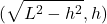.
We need equation to the line perpendicular from R to PQ. Clearly slope of that line will be negative reciprocal of the slope of PQ.
Hence it's equation is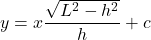where c is the y intercept. We replace x and y in this equation by the x and y coordinate of R (since we know that the line passes through R) to get the value of c..
Therefore equation to this line is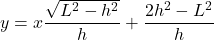.
Finally we get the coordinate of the point A by solving the following equations: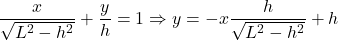Solving these two equations we will get very complicated expressions for x and y, from which it is difficult to eliminate h. So we apply a small trick. We observe the expression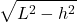. Since h may range from 0 to L, we parametrize h, and replace it by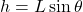. This reduces the given equations of straight line in terms of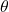. (This technique is a much used heuristic in locus problems. If you are seeing this for the first time, it may seem a little bit unfamiliar).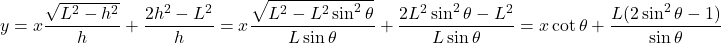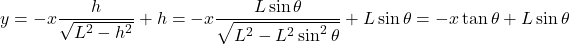To solve these two equations we equate:This implies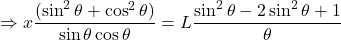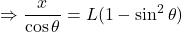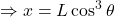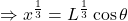Similarly we will find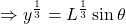Squaring and adding the last two expressions give us:## Chatuspathi:

• What is this topic: Locus Problem, Coordinate Geometry
• What are some of the associated concept: Intercept form of straight line equation, product of slopes of perpendicular lines is -1, parametrization of variable
• Where can learn these topics: Cheenta I.S.I. & C.M.I. course, discusses these topics in the ‘Coordinate Geometry’ module.
• Book Suggestions: Coordinate Geometry Volume I by S.L. Loney, Lines and Curves by Vasilyev

Problem: Problem on Asteroid

Let PQ be a line segment of a fixed length L with it's two ends P and Q sliding along the X axis and Y-axis respectively. Complete the rectangle OPRQ where O is the origin. Show that the locus of the foot of the perpendicular drawn from R on PQ is given byDiscussion:

This beautiful problem discuss locus of an asteroid.

There are several locus definition of asteroid. A good reference book for a more detailed account is 'Lines and Curves' by Vasiliev. (This book is not available in print. We have an electronic copy, which we may give you for personal use).

Here we will work only on the problem (leaving the detailed discussion for class).

We will work with the following diagram:Since length of PQ is L, if coordinate of Q = (0,h) then the coordinate of. Hence equation to line PQ is.
Coordinate of R is.
We need equation to the line perpendicular from R to PQ. Clearly slope of that line will be negative reciprocal of the slope of PQ.
Hence it's equation iswhere c is the y intercept. We replace x and y in this equation by the x and y coordinate of R (since we know that the line passes through R) to get the value of c..
Therefore equation to this line is.
Finally we get the coordinate of the point A by solving the following equations:Solving these two equations we will get very complicated expressions for x and y, from which it is difficult to eliminate h. So we apply a small trick. We observe the expression. Since h may range from 0 to L, we parametrize h, and replace it by. This reduces the given equations of straight line in terms of. (This technique is a much used heuristic in locus problems. If you are seeing this for the first time, it may seem a little bit unfamiliar).To solve these two equations we equate:This impliesSimilarly we will findSquaring and adding the last two expressions give us:## Chatuspathi:

• What is this topic: Locus Problem, Coordinate Geometry
• What are some of the associated concept: Intercept form of straight line equation, product of slopes of perpendicular lines is -1, parametrization of variable
• Where can learn these topics: Cheenta I.S.I. & C.M.I. course, discusses these topics in the ‘Coordinate Geometry’ module.
• Book Suggestions: Coordinate Geometry Volume I by S.L. Loney, Lines and Curves by Vasilyev

This site uses Akismet to reduce spam. Learn how your comment data is processed.

### Knowledge Partner